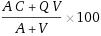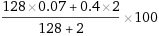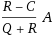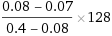## Thursday, August 15, 2019

### How Adding Bourbon Raises The ABV In Beer

Effects On ABV With Alcohol Additions

Joe “Jomebrew” Aiello

Synopsis

This is a followup to my previous article where I provided information on how to spike flavored sparkling water to make hard seltzer.

This article explains the effects on a beers ABV when you add alcohol directly to the beer. We’ll show how to calculate the change in ABV of a beer when you add something with alcohol in it such as wine or distilled spirits such as Bourbon.

For example, what is the new ABV when I add 2 ounces of Bourbon to my 7% ABV beer?

I will also explain how to calculate how much alcohol, such as wine or distilled spirit, is needed to raise the ABV of your beer to a specific ABV. For example, how much alcohol is needed to raise a beer from 7% ABV to 8% ABV.

Of course, this is not limited to beer. You can calculate the amount of alcohol in a mixed drink too. I’ll explain that later.

Find the ABV Calculator Here- Look For ABV Adjustments

Why?

To have a relatively precise understanding of how much ABV is raised when I add alcohol to a beer. When I add alcohol such wine or a spirit like bourbon to a beer to achieve a taste profile I it raised the overall OBV of the beer. For those like me, knowing the effect on the ABV is interesting and a little compulsive.

In addition to knowing the effects on ABV when adding alcohol, I’ve been interested in knowing how much alcohol is needed to raise the ABV of a beer (or other beverages) to a specific percent of alcohol.

Does This Only Work For Beer?

These formulas can be used to calculate the change or how much is needed to raise the ABV in any beverage.

Where my previous article talked about spiking a sparkling water with no existing alcohol, this article shows you how to calculate raise the ABV of a beverage that has zero or some alcohol already.  It can also be used to spike your sparkling water.

The Problem

How much is the ABV of a beverage raised when adding a distilled spirit. We’ll call this New ABV.

How much alcohol is needed to raise the ABV to a specific ABV. We’ll call this Raise ABV.

We need to start with a few bits of information. These first values are common for either formula.

First, you need to start with the volume of the beverage. For this example, I have 120 ounces of of a Baltic Porter.

Next, you need the percentage of alcohol in the baltic porter. In the example, I am using 7%. We will use .07 for the calculations to keep it simple (7/100)

Now, you need to know the percentage of alcohol (ABV) of the distilled spirit. Here, I am using 80 proof vodka which is 40% alcohol. For the calculations, we will use .4 (40/100)

For New ABV, we need to know how much alcoholic liquid are we adding. We’ll use 2 ounces in this example.

For Raise ABV, we need to know what the target ABV is. We’ll use 8% ABV.

Finally! We have all the values needed to do our calculations.

Breaking Down The Calculation for New ABV

The Formula we worked out is ((A*C)+(Q*V)) / (A+V)*100 orWhere:
A = Starting Volume
C= Current ABV of the Beverage
Q = ABV of the Alcohol Being Added
V = Volume of Alcohol Added to the Beverage

As mentioned earlier, we’ll use the decimal value of the percentages:
A = 128
C = .07
Q = .4
V = 2

So, plugging the numbers into the formula, we get ((128*.07)+(.4*2)) / (128+2)*100 orThis means that when I add 2 ounces of Bourbon at 80 proof to 1 gallon of beer, It will raise the ABV by .5% (half a percent) increasing my Baltic Porter from 7% ABV to 7.5%ABV.

Breaking Down The Calculation for Raise ABV

The Formula we worked out is (R-C) / (Q+R)*A orWhere:
A = Starting Volume
C= Current ABV of the Beverage
R = Target ABV of the Beverage
Q = ABV of the Alcohol Being Added

As mentioned earlier, we’ll use the decimal value of the percentages:
A = 128
C = .07
R = .08
Q = .4

So, plugging the numbers into the formula, we get (.08-.07) / (.4+.08)*128 orThis means to increase my 7% ABV Porter to 8% ABV, I need to add 4 ounces of 80 proof Bourbon. Sure, we knew this already since 2 ounces added .5% we can simply double that. But now we can calculate it too.

Please note: The formula for Raise ABV breaks when the ABV of the Alcohol Being Added equals the Target ABV of the Beverage. So, Don’t try to add 40% ABV liquor to achieve a 40% ABV.

How does this relate to calories?

Distilled spirits at 80 proof / 40% ABV run 64 calories per ounce. Since we are adding 2 ounces of 40% ABV distilled spirit, we simply multiply 2 X 64 which equals 128 calories are being added to a gallon of beer. So, you are adding 1 calorie per ounce or 12 calories per 12 ounces of beer plus the calories already in the beer.

How can I calculate the ABV Other Drinks?

How about a shaken Margarita? This can be tricky and you may need to run through the calculations a couple times if you are using multiple liquors with different ABV.

Take, for example a shaken Margarita. A common recipe is 2 ounces Tequila (80 proof), 1 ounce TripleSec (Dekuyper - 48 proof) and 1 ounce lime and about 1 ounce dilution from ice.

We have 5 ounces of volume.
2 ounces at 40% ABV
1 ounce at 24% ABV
1 ounce Lime at 0 ABV
1 ounces at 0 ABV

So, Add Tequila to the Ice and Lime and you have 4 ounces at 20% ABV. Now add 1 ounce Triple Sec and you have 5 ounces at 21% ABV.

That’s it. A Margarita using this recipe has about 21% ABV per 5 ounces which contains 1.05 ounces of alcohol. Compare to a 16 ounce beer at 6.5% ABV contains about the same alcohol.

Say you drop a 1 ounce shot of Jameson in your Guinness. What is the resulting ABV?

You have a 12 Ounce Guinness at 5% ABV. You add 1 ounce Jameson at 40% ABV. Plug in those values to the New ABV formula and you get a 13 ounce beer with 7.7 % ABV.

So, I dumped a 25 ounce bottle of Cabernet into my 5 gallon batch of 7% ABV Brown Ale. What is the new ABV? Plug the values into the New ABV formula and you get 7.2% ABV

How about dilution? I added 12 ounces of flavored sparkling water with 0% ABV into 2 ounces of 40% ABV Vodka. What is my ABV? Plug the values into the New ABV formula and you get 5.7% ABV.